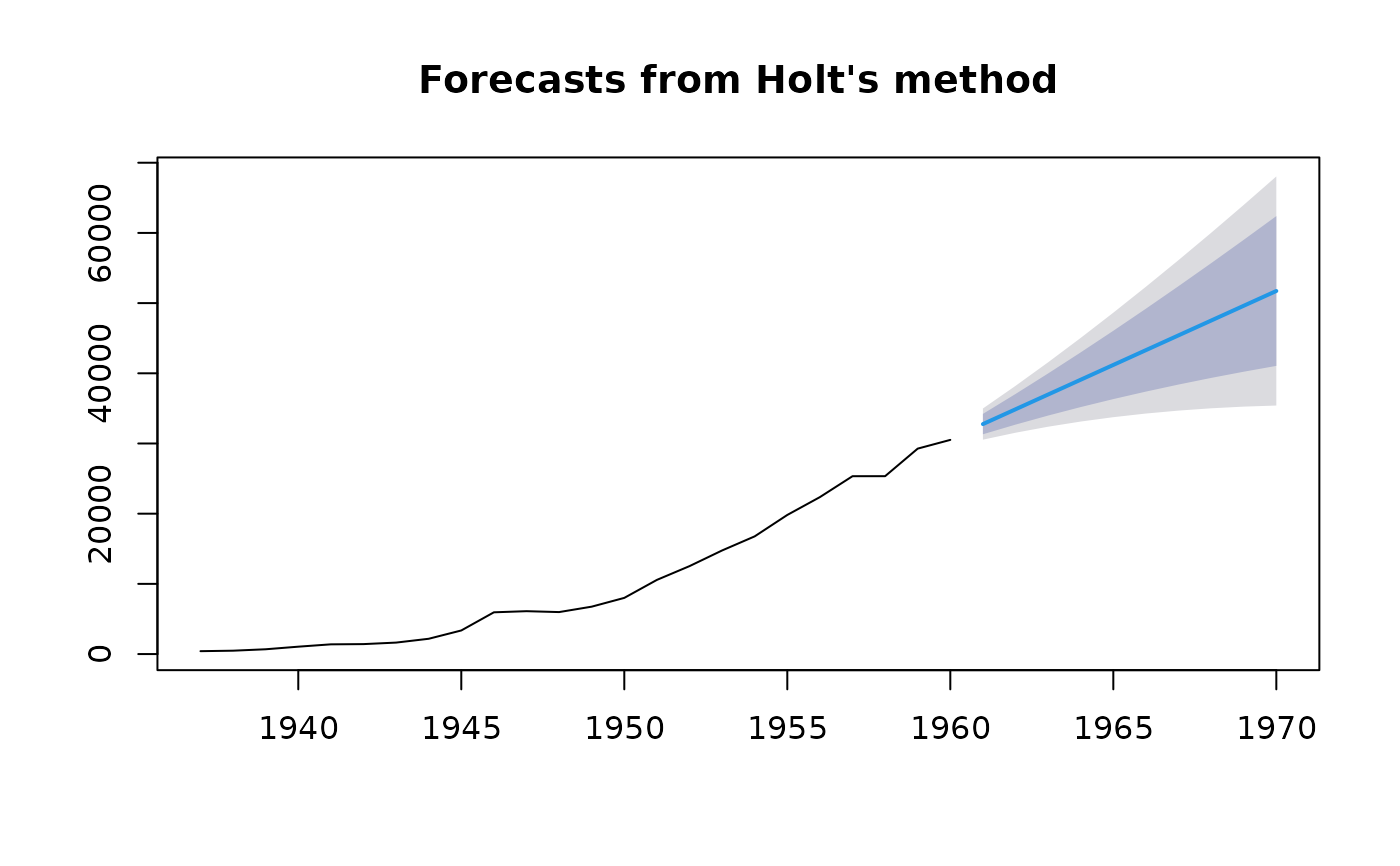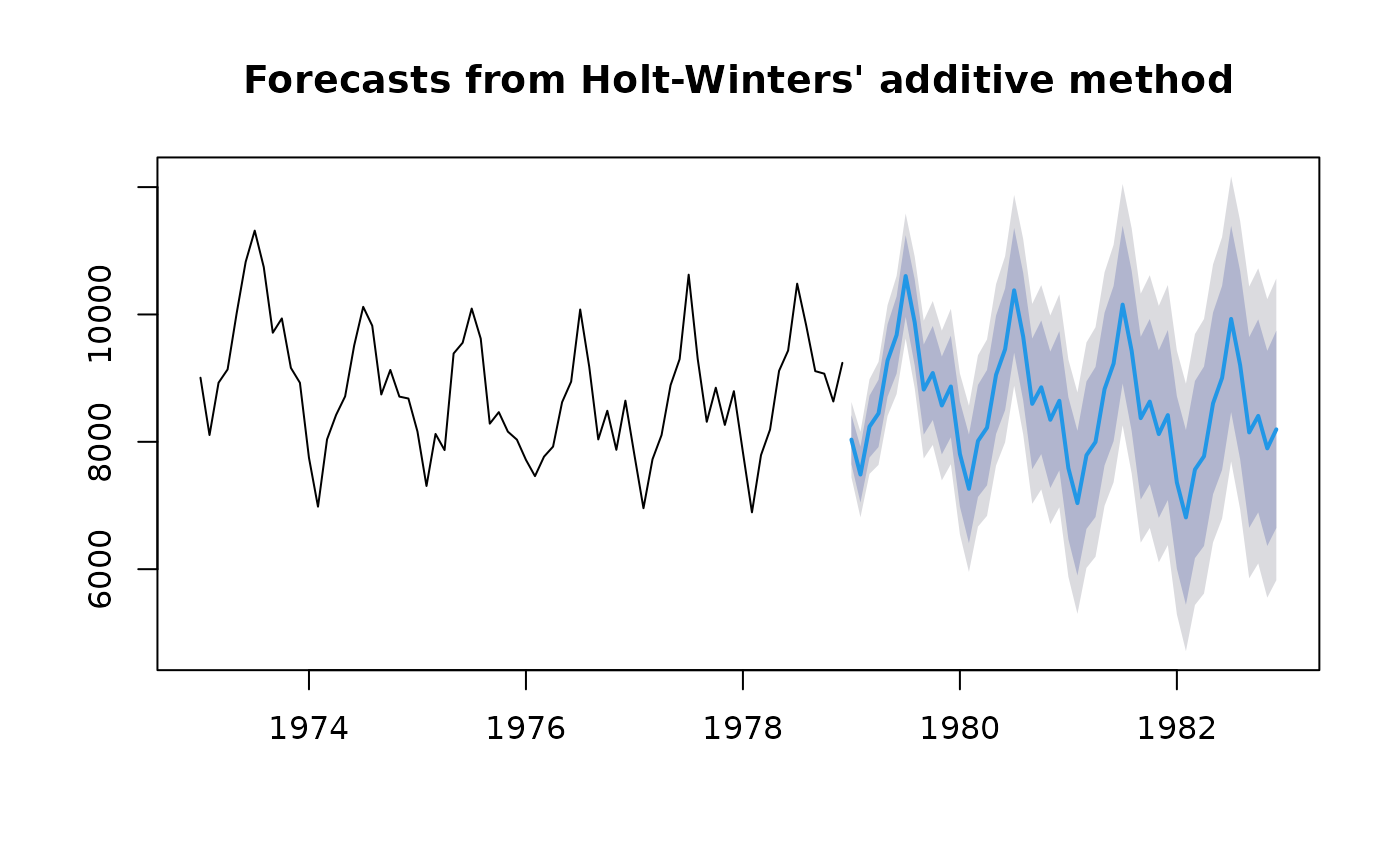Returns forecasts and other information for exponential smoothing forecasts applied to y.

ses(
y,
h = 10,
level = c(80, 95),
fan = FALSE,
initial = c("optimal", "simple"),
alpha = NULL,
lambda = NULL,
x = y,
...
)

holt(
y,
h = 10,
damped = FALSE,
level = c(80, 95),
fan = FALSE,
initial = c("optimal", "simple"),
exponential = FALSE,
alpha = NULL,
beta = NULL,
phi = NULL,
lambda = NULL,
x = y,
...
)

hw(
y,
h = 2 * frequency(x),
damped = FALSE,
level = c(80, 95),
fan = FALSE,
initial = c("optimal", "simple"),
exponential = FALSE,
alpha = NULL,
beta = NULL,
gamma = NULL,
phi = NULL,
lambda = NULL,
x = y,
...
)

## Arguments

y a numeric vector or time series of class ts Number of periods for forecasting. Confidence level for prediction intervals. If TRUE, level is set to seq(51,99,by=3). This is suitable for fan plots. Method used for selecting initial state values. If optimal, the initial values are optimized along with the smoothing parameters using ets. If simple, the initial values are set to values obtained using simple calculations on the first few observations. See Hyndman & Athanasopoulos (2014) for details. Value of smoothing parameter for the level. If NULL, it will be estimated. Box-Cox transformation parameter. If lambda="auto", then a transformation is automatically selected using BoxCox.lambda. The transformation is ignored if NULL. Otherwise, data transformed before model is estimated. Use adjusted back-transformed mean for Box-Cox transformations. If transformed data is used to produce forecasts and fitted values, a regular back transformation will result in median forecasts. If biasadj is TRUE, an adjustment will be made to produce mean forecasts and fitted values. Deprecated. Included for backwards compatibility. Other arguments passed to forecast.ets. If TRUE, use a damped trend. If TRUE, an exponential trend is fitted. Otherwise, the trend is (locally) linear. Value of smoothing parameter for the trend. If NULL, it will be estimated. Value of damping parameter if damped=TRUE. If NULL, it will be estimated. Type of seasonality in hw model. "additive" or "multiplicative" Value of smoothing parameter for the seasonal component. If NULL, it will be estimated.

## Value

An object of class "forecast".

The function summary is used to obtain and print a summary of the results, while the function plot produces a plot of the forecasts and prediction intervals.

The generic accessor functions fitted.values and residuals extract useful features of the value returned by ets and associated functions.

An object of class "forecast" is a list containing at least the following elements:

model

A list containing information about the fitted model

method

The name of the forecasting method as a character string

mean

Point forecasts as a time series

lower

Lower limits for prediction intervals

upper

Upper limits for prediction intervals

level

The confidence values associated with the prediction intervals

x

The original time series (either object itself or the time series used to create the model stored as object).

residuals

Residuals from the fitted model.

fitted

Fitted values (one-step forecasts)

## Details

ses, holt and hw are simply convenient wrapper functions for forecast(ets(...)).

Hyndman, R.J., Koehler, A.B., Ord, J.K., Snyder, R.D. (2008) Forecasting with exponential smoothing: the state space approach, Springer-Verlag: New York. http://www.exponentialsmoothing.net.

Hyndman and Athanasopoulos (2018) Forecasting: principles and practice, 2nd edition, OTexts: Melbourne, Australia. https://otexts.com/fpp2/

ets, HoltWinters, rwf, arima.

Rob J Hyndman

## Examples


fcast <- holt(airmiles)
plot(fcast)deaths.fcast <- hw(USAccDeaths,h=48)
plot(deaths.fcast)